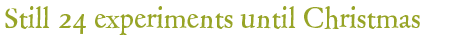## Experiment of December 8, 2021:

The time to submit an answer is over. You can now view the solution of the experiment.

## Question:

What can Ms. Santa read from the recording of the bouncing ball?

A) The ball height decreases, the proportional energy loss in % remains about the same.

B) The ball height decreases, the proportional energy loss in % increases.

C) The ball height decreases, the proportional energy loss in % decreases.

D) The ball height remains the same, the proportional energy loss in % increases.